码字不易，转载请注明出处~
本文来源于下面博客：
前面讲到梯度下降法以及带动量的梯度下降法、NAG等，它们的一个共同的特点是都包含学习率$\eta$这个参数，而这个参数的选择属于超参数的范围。个人感觉超参数包含了两方面的内容：1. 参数需要人工调整，不是一次设置之后系统自动处理；2. 对全局的影响相当大，不同的取值在一定程度上影响整体的效果。然而超参数的麻烦之处在于，它是需要人不断调整试优的，或大或小都可能导致网络无法达到最优解。更麻烦的是，对于神经网络而言，它本身需要解决的是一个高维的非凸优化问题，维度高达上百万维，极有可能在不同的维度上，某个参数比较适合而在另外一些维度上面，该参数就可能不适合了。对于学习率，面临的就是这种情况。因此，我们希望最好的情况是学习率能够根据网络训练样本的变化而自动调整，这样就省去了很多人为的工作，更重要的是，这样也许能够使网络自己找到最好的优化方向，而不是去地毯式搜索。
该算法应对的就是在高维空间中，网络学习率在各个维度上面应该如何变化这个问题。公式如下：
$\theta_{t+1}=\theta_t-\frac{\eta}{\sqrt{\varepsilon I+diag(G_t)}}\cdot g_t\tag{1}$
式中，$\theta$是需要更新的权重参数，$\eta$是初始化学习率，$\varepsilon$是为了避免除0引入的因子，$I$是单位矩阵，$g_t$是在t时刻所计算得到的梯度，公式如下：
$g_t=\frac{1}{n}\sum_{i=1}^{n}\nabla_\theta L(x^{(i)}, y{(i)}, \theta_t)\tag{2}$
最关键的是$G_t$矩阵，它表示到t时刻为止，所有之前和现在所累积的梯度信息，公式如下：
$G_t=\sum_{\tau=1}^{t}g_\tau g_{\tau}^{T}\tag{3}$
看着有点累人，感觉每次计算都要计算一个大矩阵。实际上，也的确如此。如果每次使用$G_t$进行计算，那么势必会有很大的计算量，更何况还要进行矩阵的开方运算。所以，如果我们仅仅计算对角化的$G_t$，情况就会好很多，毕竟对角阵有很好的性质，所有的操作都可以应用到每一个对角元素上面而无需增加额外的运算负担。这样就可以很容易进行参数更新了。
$\begin{bmatrix} \theta_{t+1}^{(1)} \\ \theta_{t+1}^{(2)} \\ \vdots \\ \theta_{t+1}^{(m)}\end{bmatrix}=\begin{bmatrix} \theta_t^{1)} \\ \theta_t^{(2)} \\ \vdots \\ \theta_t^{(m)}\end{bmatrix}-\eta {\begin{pmatrix}{\begin{bmatrix}&\varepsilon &0 &\cdots &0 \\ &0 &\varepsilon &\cdots &0 \\ &\vdots &\vdots &\ddots &\vdots \\ &0 &0 &\cdots &\varepsilon \end{bmatrix}}+\begin{bmatrix}&G_t^{(1, 1)} &0 &\cdots &0\\ &0 &G_t^{(2, 2)} &\cdots &0 \\ &\vdots &\vdots &\ddots &\vdots \\ &0 &0 &\cdots &G_t^{m, m} \end{bmatrix}\end{pmatrix}}^{-1/2} \cdot \begin{bmatrix} &g_t^{(1)}\\ &g_t^{(2)} \\&\vdots \\&g_t^{(m)} \end{bmatrix}$
还是看起来挺复杂的，其实只需要关注的是学习率更新这部分内容，所有可以进行适当的简化，就可以得到如下的式子：
$\begin{bmatrix} \theta_{t+1}^{(1)} \\ \theta_{t+1}^{(2)} \\ \vdots \\ \theta_{t+1}^{(m)} \end{bmatrix}= \begin{bmatrix} \theta_t^{1)} \\ \theta_t^{(2)} \\ \vdots \\ \theta_t^{(m)} \end{bmatrix} \begin{bmatrix} &\frac{\eta}{\sqrt{\varepsilon+G_t^{(1,1)}}} &0 &\cdots &0 \\ &0 &\frac{\eta}{\sqrt{\varepsilon+G_t^{(2,2)}}} &\cdots &0 \\ &\vdots &\vdots &\ddots &\vdots \\ &0 &0 &\cdots &\frac{\eta}{\sqrt{\varepsilon+G_t^{(m,m)}}}\end{bmatrix} \cdot \begin{bmatrix} &g_t^{(1)}\\ &g_t^{(2)} \\ &\vdots \\&g_t^{(m)} \end{bmatrix}$
$\begin{bmatrix} \theta_{t+1}^{(1)} \\ \theta_{t+1}^{(2)} \\ \vdots \\ \theta_{t+1}^{(m)} \end{bmatrix}= \begin{bmatrix} \theta_t^{1)} \\ \theta_t^{(2)} \\ \vdots \\ \theta_t^{(m)} \end{bmatrix} \begin{bmatrix} &\frac{\eta}{\sqrt{\varepsilon+G_t^{(1,1)}}}g_t^{(1)} \\ &\frac{\eta}{\sqrt{\varepsilon+G_t^{(2,2)}}}g_t^{(2)}\\ &\vdots \\ &\frac{\eta}{\sqrt{\varepsilon+G_t^{(m,m)}}}g_t^{(m)}\end{bmatrix} \tag{4}$
对比之前文章中的随机梯度下降法：
$\begin{bmatrix} \theta_{t+1}^{(1)} \\ \theta_{t+1}^{(2)} \\ \vdots \\ \theta_{t+1}^{(m)} \end{bmatrix}= \begin{bmatrix} \theta_t^{1)} \\ \theta_t^{(2)} \\ \vdots \\ \theta_t^{(m)} \end{bmatrix} \begin{bmatrix} &\eta g_t^{(1)} \\ &\eta g_t^{(2)} \\ &\vdots \\ &\eta g_t^{(m)} \\ \end{bmatrix} \tag{5}$
可以看到，这里相当于每次在进行参数更新的时候，将$\eta$缩放了$\frac{1}{\sqrt(\varepsilon+G_t)}$倍，而且由于$G_t$每次都累加梯度，因此是发生变化的。这就看上去仿佛学习率是发生变化的。虽然事实上只是梯度$g_t$之前的因子发生了变化，我觉得这里应该理解为，学习率不变，只不过给学习率乘以了一个变化的变量，因此看上去好像学习率发生了变化。当然，如果把梯度之前的乘子看做是学习率，那理解为学习率变化也没什么问题。所以，AdaGrad的做法就是这样。
实际上，(3)中的公式也不太好理解，如果写成下面这样或许更直白易懂：
$G_t^{(i,i)}= \sum_{\tau=1}^{t}(g_\tau^{(i)})^2\tag{6}$
那么参数的更新过程就可以写作：
$\theta_{t+1}=\theta_t-\frac{\eta}{\sqrt{\varepsilon +\sum{g_t^2}}}\bigodot g_t\tag{7}$
式中的矩阵乘积用了$\bigodot$。之所以(3)写成那样，主要是为了理解实际上真实发生的是矩阵的的操作，而且缩放因子中的$G$代表的是对角矩阵。
算法对于稀疏的数据所表现的性能更好，对于经常出现的特征，我们希望他们他们的学习率更新不要太快，而对不常出现的特征，则希望学习率更新快一些，从而能够让网络学习到的特征更好。但由于一般我们在网络学习之初就对每一次梯度进行了累积，所以当网络训练到一定程度的时候（不一定收敛），很容易导致网络没法继续学习，因为此时的学习率接近0。而这个问题需要进行进一步改进，也就是下面其他的自适应学习率方法所做的工作。
参考

J. Duchi, E. Hazan, Y. Singer, “Adaptive Subgradient Methods for Online Learning and Stochastic Optimization” (2011)
I. Goodfellow, Y. Bengio, A. Courville, “Deep Learning” (2016)


展开全文深度学习 优化方法

千次阅读 2019-08-02 17:04:52
参考：

表示第i个参数的梯度，对于经典的SGD优化方法，参数θ的更新为：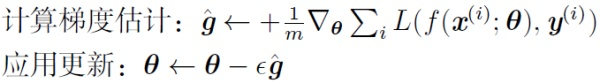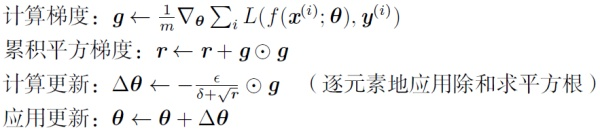其中，r为梯度累积变量，r的初始值为0。ε为全局学习率，需要自己设置。δ为小常数，为了数值稳定大约设置为。

优势：

在数据分布稀疏的场景，能更好利用稀疏梯度的信息，比标准的SGD算法更有效地收敛。

缺点：

主要缺陷来自分母项的对梯度平方不断累积，随之时间步地增加，分母项越来越大，最终导致学习率收缩到太小无法进行有效更新。

展开全文学习衰减率：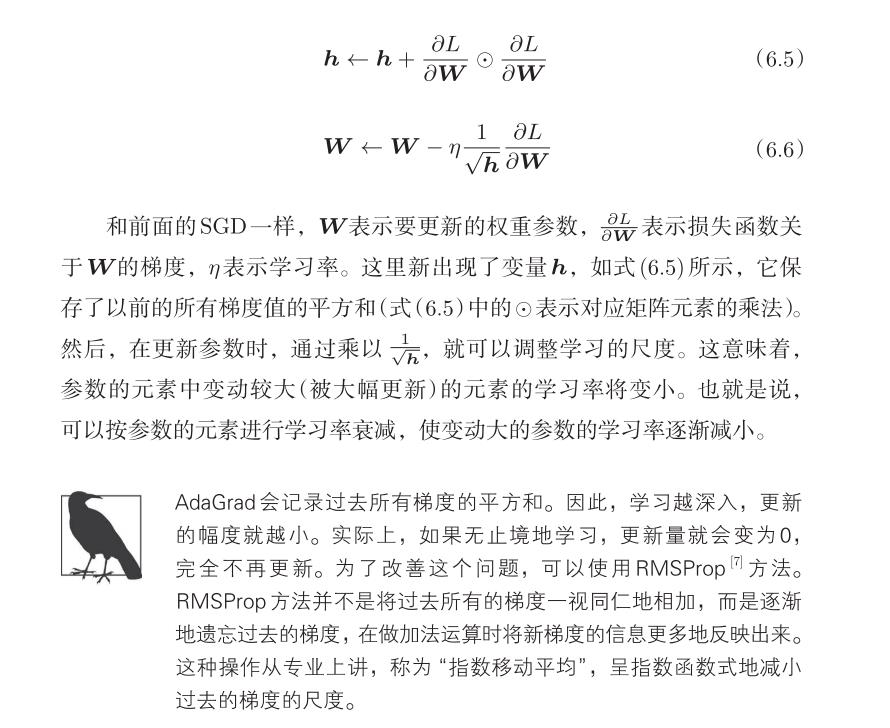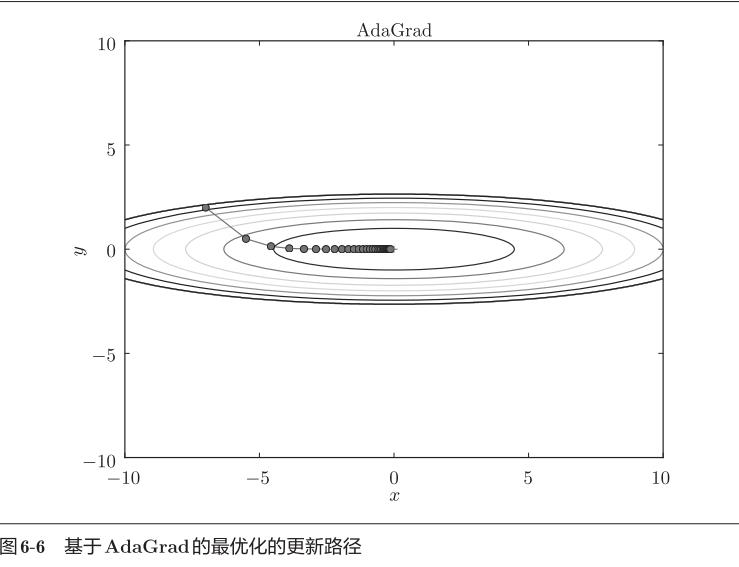# coding： utf-8
import numpy as np

def __init__(self, learning_rate=0.01):
self.learning_rate = learning_rate
self.h = None

if self.h is None:
self.h = {}
for key, value in params.items():
self.h[key] = np.zeros_like(value)

for key in params.keys():
params[key] -= self.learning_rate * grads[key] / (np.sqrt(self.h[key]) + 1e-07) # 1e-07微小值避免分母为0


展开全文• “微信公众号” 梯度下降算法、随机梯度下降算法（SGD）、小批量梯度下降算法（mini-batch SGD）、动量法（momentum）、Nesterov动量法有一个共同的特点是：对于每一个参数都用相同的学习率进行更新。...
“微信公众号”本文文章同步更新在我的知乎专栏里面：深度学习优化方法-AdaGrad - Microstrong的文章 - 知乎https://zhuanlan.zhihu.com/p/38298197        梯度下降算法、随机梯度下降算法（SGD）、小批量梯度下降算法（mini-batch SGD）、动量法（momentum）、Nesterov动量法有一个共同的特点是：对于每一个参数都用相同的学习率进行更新。       但是在实际应用中，各个参数的重要性肯定是不一样的，所以我们对于不同的参数要动态的采取不同的学习率，让目标函数更快的收敛。       AdaGrad算法就是将每一个参数的每一次迭代的梯度取平方累加后在开方，用全局学习率除以这个数，作为学习率的动态更新。AdaGrad算法介绍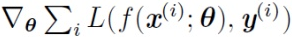表示第i个参数的梯度，对于经典的SGD优化方法，参数θth的更新为：再来看AdaGrad算法表示为：其中，r为梯度累积变量，r的初始值为0。ε为全局学习率，需要自己设置。δ为小常数，为了数值稳定大约设置为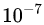。AdaGrad算法分析 （1）从AdaGrad算法中可以看出，随着算法不断迭代，r会越来越大，整体的学习率会越来越小。所以，一般来说AdaGrad算法一开始是激励收敛，到了后面就慢慢变成惩罚收敛，速度越来越慢。（2）在SGD中，随着梯度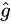的增大，我们的学习步长应该是增大的。但是在AdaGrad中，随着梯度g的增大，我们的r也在逐渐的增大，且在梯度更新时r在分母上，也就是整个学习率是减少的，这是为什么呢？这是因为随着更新次数的增大，我们希望学习率越来越慢。因为我们认为在学习率的最初阶段，我们距离损失函数最优解还很远，随着更新次数的增加，越来越接近最优解，所以学习率也随之变慢。（3）经验上已经发现，对于训练深度神经网络模型而言，从训练开始时积累梯度平方会导致有效学习率过早和过量的减小。AdaGrade在某些深度学习模型上效果不错，但不是全部。Reference【1】深度学习，Ian Goodfellow / Yoshua Bengio所著。【2】深度学习优化函数详解（6）-- adagrad【3】adagrad原理
展开全文深度学习 神经网络 优化算法

千次阅读 多人点赞 2020-08-10 12:38:59深度学习 机器学习 人工智能 pytorch

千次阅读 2018-12-30 22:04:12

千次阅读 2019-06-02 20:53:25深度学习 人工智能 机器学习
• 思想：如果一个参数的梯度一直都非常大，就让它的学习率变小一点，防止震动，反之，则让其学习率变大，使其能更快更新 做法：学习率由下列式子所得 +后的参数是为了防止分母等于0，一般取10的-10次方 ...
• 在之前介绍过的优化算法中，⽬标函数⾃变量的每一个元素在相同时间步都使用同一个学习率来⾃我迭代。举个例子，假设⽬标函数为f，⾃变量为一个二维向量,该向量中每一个元素在迭代时都使⽤相同的学习率。例如，在学习...动手学PyTorch
• 1. 稀疏特征和学习率 在模型训练中会有稀疏特征（很少发生的特征），在自然语言模型中常见，比如，与 “学习”相比“预处理”更加少见。同时在其他领域也会用到，例如计算广告和个性化的协同过滤。...深度学习 mxnetRMSProp AdaDelta优化 机器学习 深度学习深度学习RMSprop 自适应学习率matlab RMSProp
• 深度学习优化函数详解系列目录 深度学习优化函数详解（0）– 线性回归问题 深度学习优化函数详解（1）– Gradient Descent 梯度下降法 深度学习优化函数详解（2）– SGD 随机梯度下降 深度学习优化函数详解...深度学习
• 1. SGDBatch Gradient Descent在每一轮的训练过程中，Batch Gradient Descent算法用整个训练集的数据计算cost fuction的梯度，并用该梯度对模型参数进行更新：Θ=Θ−α⋅▽ΘJ(Θ)\Theta = \Theta -\alpha \cdot \...Momentum神经网络 深度学习深度学习 python 人工智能 机器学习 神经网络